## Use sum and difference formulas for cosine

Finding the exact value of the sine, cosine, or tangent of an angle is often easier if we can rewrite the given angle in terms of two angles that have known trigonometric values. We can use the special angles, which we can review in the unit circle shown in Figure 2.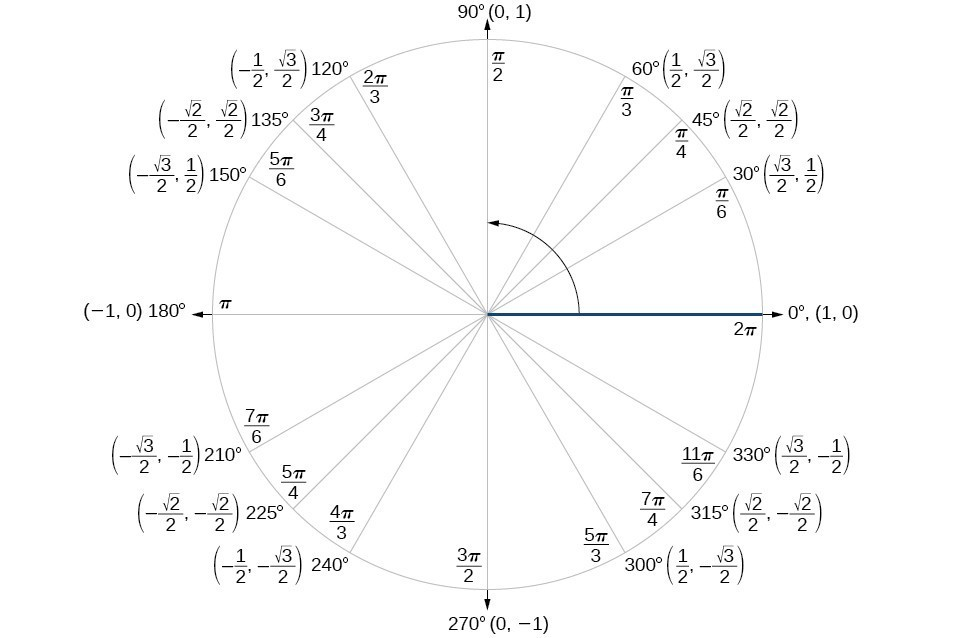Figure 2. The Unit Circle

We will begin with the sum and difference formulas for cosine, so that we can find the cosine of a given angle if we can break it up into the sum or difference of two of the special angles.

 Sum formula for cosine $\cos \left(\alpha +\beta \right)=\cos \alpha \cos \beta -\sin \alpha \sin \beta$ Difference formula for cosine $\cos \left(\alpha -\beta \right)=\cos \alpha \cos \beta +\sin \alpha \sin \beta$

First, we will prove the difference formula for cosines. Let’s consider two points on the unit circle. Point $P$ is at an angle $\alpha$ from the positive x-axis with coordinates $\left(\cos \alpha ,\sin \alpha \right)$ and point $Q$ is at an angle of $\beta$ from the positive x-axis with coordinates $\left(\cos \beta ,\sin \beta \right)$. Note the measure of angle $POQ$ is $\alpha -\beta$.

Label two more points: $A$ at an angle of $\left(\alpha -\beta \right)$ from the positive x-axis with coordinates $\left(\cos \left(\alpha -\beta \right),\sin \left(\alpha -\beta \right)\right)$; and point $B$ with coordinates $\left(1,0\right)$. Triangle $POQ$ is a rotation of triangle $AOB$ and thus the distance from $P$ to $Q$ is the same as the distance from $A$ to $B$.

Figure 3. We can find the distance from $P$ to $Q$ using the distance formula.

$\begin{array}{l}{d}_{PQ}=\sqrt{{\left(\cos \alpha -\cos \beta \right)}^{2}+{\left(\sin \alpha -\sin \beta \right)}^{2}}\hfill \\ \text{ }=\sqrt{{\cos }^{2}\alpha -2\cos \alpha \cos \beta +{\cos }^{2}\beta +{\sin }^{2}\alpha -2\sin \alpha \sin \beta +{\sin }^{2}\beta }\hfill \end{array}$

Then we apply the Pythagorean identity and simplify.

$\begin{array}{l}\begin{array}{l}\hfill \\ \hfill \\ =\sqrt{\left({\cos }^{2}\alpha +{\sin }^{2}\alpha \right)+\left({\cos }^{2}\beta +{\sin }^{2}\beta \right)-2\cos \alpha \cos \beta -2\sin \alpha \sin \beta }\hfill \end{array}\hfill \\ =\sqrt{1+1 - 2\cos \alpha \cos \beta -2\sin \alpha \sin \beta }\hfill \\ =\sqrt{2 - 2\cos \alpha \cos \beta -2\sin \alpha \sin \beta }\hfill \end{array}$

Similarly, using the distance formula we can find the distance from $A$ to $B$.

$\begin{array}{l}{d}_{AB}=\sqrt{{\left(\cos \left(\alpha -\beta \right)-1\right)}^{2}+{\left(\sin \left(\alpha -\beta \right)-0\right)}^{2}}\hfill \\ \text{ }=\sqrt{{\cos }^{2}\left(\alpha -\beta \right)-2\cos \left(\alpha -\beta \right)+1+{\sin }^{2}\left(\alpha -\beta \right)}\hfill \end{array}$

Applying the Pythagorean identity and simplifying we get:

$\begin{array}{l}\begin{array}{l}\hfill \\ \hfill \\ =\sqrt{\left({\cos }^{2}\left(\alpha -\beta \right)+{\sin }^{2}\left(\alpha -\beta \right)\right)-2\cos \left(\alpha -\beta \right)+1}\hfill \end{array}\hfill \\ =\sqrt{1 - 2\cos \left(\alpha -\beta \right)+1}\hfill \\ =\sqrt{2 - 2\cos \left(\alpha -\beta \right)}\hfill \end{array}$

Because the two distances are the same, we set them equal to each other and simplify.

$\begin{array}{l}\begin{array}{l}\hfill \\ \sqrt{2 - 2\cos \alpha \cos \beta -2\sin \alpha \sin \beta }=\sqrt{2 - 2\cos \left(\alpha -\beta \right)}\hfill \end{array}\hfill \\ \text{ }2 - 2\cos \alpha \cos \beta -2\sin \alpha \sin \beta =2 - 2\cos \left(\alpha -\beta \right)\text{ }\hfill \end{array}$

Finally we subtract $2$ from both sides and divide both sides by $-2$.

$\cos \alpha \cos \beta +\sin \alpha \sin \beta =\cos \left(\alpha -\beta \right)\text{ }$

Thus, we have the difference formula for cosine. We can use similar methods to derive the cosine of the sum of two angles.

### A General Note: Sum and Difference Formulas for Cosine

These formulas can be used to calculate the cosine of sums and differences of angles.

$\cos \left(\alpha +\beta \right)=\cos \alpha \cos \beta -\sin \alpha \sin \beta$
$\cos \left(\alpha -\beta \right)=\cos \alpha \cos \beta +\sin \alpha \sin \beta$

### How To: Given two angles, find the cosine of the difference between the angles.

1. Write the difference formula for cosine.
2. Substitute the values of the given angles into the formula.
3. Simplify.

### Example 1: Finding the Exact Value Using the Formula for the Cosine of the Difference of Two Angles

Using the formula for the cosine of the difference of two angles, find the exact value of $\cos \left(\frac{5\pi }{4}-\frac{\pi }{6}\right)$.

### Solution

Use the formula for the cosine of the difference of two angles. We have

$\begin{array}{l}\hfill \\ \begin{array}{l}\text{ }\cos \left(\alpha -\beta \right)=\cos \alpha \cos \beta +\sin \alpha \sin \beta \hfill \\ \cos \left(\frac{5\pi }{4}-\frac{\pi }{6}\right)=\cos \left(\frac{5\pi }{4}\right)\cos \left(\frac{\pi }{6}\right)+\sin \left(\frac{5\pi }{4}\right)\sin \left(\frac{\pi }{6}\right)\hfill \\ \text{ }=\left(-\frac{\sqrt{2}}{2}\right)\left(\frac{\sqrt{3}}{2}\right)-\left(\frac{\sqrt{2}}{2}\right)\left(\frac{1}{2}\right)\hfill \\ \text{ }=-\frac{\sqrt{6}}{4}-\frac{\sqrt{2}}{4}\hfill \\ \text{ }=\frac{-\sqrt{6}-\sqrt{2}}{4}\hfill \\ \hfill \end{array}\hfill \end{array}$

### Try It 1

Find the exact value of $\cos \left(\frac{\pi }{3}-\frac{\pi }{4}\right)$.

Solution

### Example 2: Finding the Exact Value Using the Formula for the Sum of Two Angles for Cosine

Find the exact value of $\cos \left({75}^{\circ }\right)$.

### Solution

As ${75}^{\circ }={45}^{\circ }+{30}^{\circ }$, we can evaluate $\cos \left({75}^{\circ }\right)$ as $\cos \left({45}^{\circ }+{30}^{\circ }\right)$. Thus,

$\begin{array}{l}\cos \left({45}^{\circ }+{30}^{\circ }\right)=\cos \left({45}^{\circ }\right)\cos \left({30}^{\circ }\right)-\sin \left({45}^{\circ }\right)\sin \left({30}^{\circ }\right)\hfill \\ \text{ }=\frac{\sqrt{2}}{2}\left(\frac{\sqrt{3}}{2}\right)-\frac{\sqrt{2}}{2}\left(\frac{1}{2}\right)\hfill \\ \text{ }=\frac{\sqrt{6}}{4}-\frac{\sqrt{2}}{4}\hfill \\ \text{ }=\frac{\sqrt{6}-\sqrt{2}}{4}\hfill \end{array}$

### Try It 2

Find the exact value of $\cos \left({105}^{\circ }\right)$.

Solution

## Use sum and difference formulas for sine

The sum and difference formulas for sine can be derived in the same manner as those for cosine, and they resemble the cosine formulas.

### A General Note: Sum and Difference Formulas for Sine

These formulas can be used to calculate the sines of sums and differences of angles.

$\sin \left(\alpha +\beta \right)=\sin \alpha \cos \beta +\cos \alpha \sin \beta$
$\sin \left(\alpha -\beta \right)=\sin \alpha \cos \beta -\cos \alpha \sin \beta$

### How To: Given two angles, find the sine of the difference between the angles.

1. Write the difference formula for sine.
2. Substitute the given angles into the formula.
3. Simplify.

### Example 3: Using Sum and Difference Identities to Evaluate the Difference of Angles

Use the sum and difference identities to evaluate the difference of the angles and show that part a equals part b.

1. $\sin \left({45}^{\circ }-{30}^{\circ }\right)$
2. $\sin \left({135}^{\circ }-{120}^{\circ }\right)$

### Solution

1. Let’s begin by writing the formula and substitute the given angles.
$\begin{array}{l}\text{ }\sin \left(\alpha -\beta \right)=\sin \alpha \cos \beta -\cos \alpha \sin \beta \hfill \\ \sin \left({45}^{\circ }-{30}^{\circ }\right)=\sin \left({45}^{\circ }\right)\cos \left({30}^{\circ }\right)-\cos \left({45}^{\circ }\right)\sin \left({30}^{\circ }\right)\hfill \end{array}$

Next, we need to find the values of the trigonometric expressions.

$\sin \left({45}^{\circ }\right)=\frac{\sqrt{2}}{2},\text{ }\cos \left({30}^{\circ }\right)=\frac{\sqrt{3}}{2},\text{ }\cos \left({45}^{\circ }\right)=\frac{\sqrt{2}}{2},\text{ }\sin \left({30}^{\circ }\right)=\frac{1}{2}$

Now we can substitute these values into the equation and simplify.

$\begin{array}{l}\hfill \\ \sin \left({45}^{\circ }-{30}^{\circ }\right)=\frac{\sqrt{2}}{2}\left(\frac{\sqrt{3}}{2}\right)-\frac{\sqrt{2}}{2}\left(\frac{1}{2}\right)\hfill \\ \text{ }=\frac{\sqrt{6}-\sqrt{2}}{4}\hfill \end{array}$
2. Again, we write the formula and substitute the given angles.
$\begin{array}{l}\text{ }\sin \left(\alpha -\beta \right)=\sin \alpha \cos \beta -\cos \alpha \sin \beta \hfill \\ \sin \left({135}^{\circ }-{120}^{\circ }\right)=\sin \left({135}^{\circ }\right)\cos \left({120}^{\circ }\right)-\cos \left({135}^{\circ }\right)\sin \left({120}^{\circ }\right)\hfill \end{array}$

Next, we find the values of the trigonometric expressions.

$\sin \left({135}^{\circ }\right)=\frac{\sqrt{2}}{2},\cos \left({120}^{\circ }\right)=-\frac{1}{2},\cos \left({135}^{\circ }\right)=\frac{\sqrt{2}}{2},\sin \left({120}^{\circ }\right)=\frac{\sqrt{3}}{2}$

Now we can substitute these values into the equation and simplify.

$\begin{array}{l}\sin \left({135}^{\circ }-{120}^{\circ }\right)=\frac{\sqrt{2}}{2}\left(-\frac{1}{2}\right)-\left(-\frac{\sqrt{2}}{2}\right)\left(\frac{\sqrt{3}}{2}\right)\hfill \\ \text{ }=\frac{-\sqrt{2}+\sqrt{6}}{4}\hfill \\ \text{ }=\frac{\sqrt{6}-\sqrt{2}}{4}\hfill \\ \hfill \\ \sin \left({135}^{\circ }-{120}^{\circ }\right)=\frac{\sqrt{2}}{2}\left(-\frac{1}{2}\right)-\left(-\frac{\sqrt{2}}{2}\right)\left(\frac{\sqrt{3}}{2}\right)\hfill \\ \text{ }=\frac{-\sqrt{2}+\sqrt{6}}{4}\hfill \\ \text{ }=\frac{\sqrt{6}-\sqrt{2}}{4}\hfill \end{array}$

### Example 4: Finding the Exact Value of an Expression Involving an Inverse Trigonometric Function

Find the exact value of $\sin \left({\cos }^{-1}\frac{1}{2}+{\sin }^{-1}\frac{3}{5}\right)$.

### Solution

The pattern displayed in this problem is $\sin \left(\alpha +\beta \right)$. Let $\alpha ={\cos }^{-1}\frac{1}{2}$ and $\beta ={\sin }^{-1}\frac{3}{5}$. Then we can write

$\begin{array}{l}\hfill \\ \cos \alpha =\frac{1}{2},0\le \alpha \le \pi \hfill \\ \sin \beta =\frac{3}{5},-\frac{\pi }{2}\le \beta \le \frac{\pi }{2}\hfill \end{array}$

We will use the Pythagorean identities to find $\sin \alpha$ and $\cos \beta$.

$\begin{array}{l}\\ \\ \begin{array}{l}\sin \alpha =\sqrt{1-{\cos }^{2}\alpha }\hfill \\ \text{ }=\sqrt{1-\frac{1}{4}}\hfill \\ \text{ }=\sqrt{\frac{3}{4}}\hfill \\ \text{ }=\frac{\sqrt{3}}{2}\hfill \\ \cos \beta =\sqrt{1-{\sin }^{2}\beta }\hfill \\ \text{ }=\sqrt{1-\frac{9}{25}}\hfill \\ \text{ }=\sqrt{\frac{16}{25}}\hfill \\ \text{ }=\frac{4}{5}\hfill \end{array}\end{array}$

Using the sum formula for sine,

$\begin{array}{l}\sin \left({\cos }^{-1}\frac{1}{2}+{\sin }^{-1}\frac{3}{5}\right)=\sin \left(\alpha +\beta \right)\hfill \\ \text{ }=\sin \alpha \cos \beta +\cos \alpha \sin \beta \hfill \\ \text{ }=\frac{\sqrt{3}}{2}\cdot \frac{4}{5}+\frac{1}{2}\cdot \frac{3}{5}\hfill \\ \text{ }=\frac{4\sqrt{3}+3}{10}\hfill \end{array}$

## Use sum and difference formulas for tangent

Finding exact values for the tangent of the sum or difference of two angles is a little more complicated, but again, it is a matter of recognizing the pattern.

Finding the sum of two angles formula for tangent involves taking quotient of the sum formulas for sine and cosine and simplifying. Recall, $\tan x=\frac{\sin x}{\cos x},\cos x\ne 0$.

Let’s derive the sum formula for tangent.

$\begin{array}{ll}\tan \left(\alpha +\beta \right)=\frac{\sin \left(\alpha +\beta \right)}{\cos \left(\alpha +\beta \right)}\hfill & \hfill \\ \text{ }=\frac{\sin \alpha \cos \beta +\cos \alpha \sin \beta }{\cos \alpha \cos \beta -\sin \alpha \sin \beta }\hfill & \hfill \\ \text{ }=\frac{\frac{\sin \alpha \cos \beta +\cos \alpha \sin \beta }{\cos \alpha \cos \beta }}{\frac{\cos \alpha \cos \beta -\sin \alpha \sin \beta }{\cos \alpha \cos \beta }} \hfill & \text{Divide the numerator and denominator by cos}\alpha \text{cos}\beta \hfill \\ \text{ }=\frac{\frac{\sin \alpha \overline{)\cos \beta }}{\cos \alpha \overline{)\cos \beta }}+\frac{\overline{)\cos \alpha }\sin \beta }{\overline{)\cos \alpha }\cos \beta }}{\frac{\overline{)\cos \alpha }\overline{)\cos \beta }}{\overline{)\cos \alpha }\overline{)\cos \beta }}-\frac{\sin \alpha \sin \beta }{\cos \alpha \cos \beta }}\hfill & \hfill \\ \text{ }=\frac{\frac{\sin \alpha }{\cos \alpha }+\frac{\sin \beta }{\cos \beta }}{1-\frac{\sin \alpha \sin \beta }{\cos \alpha \cos \beta }}\hfill & \hfill \\ \text{ }=\frac{\tan \alpha +\tan \beta }{1-\tan \alpha \tan \beta }\hfill & \hfill \end{array}$

We can derive the difference formula for tangent in a similar way.

### A General Note: Sum and Difference Formulas for Tangent

The sum and difference formulas for tangent are:

$\tan \left(\alpha +\beta \right)=\frac{\tan \alpha +\tan \beta }{1-\tan \alpha \tan \beta }$
$\tan \left(\alpha -\beta \right)=\frac{\tan \alpha -\tan \beta }{1+\tan \alpha \tan \beta }$

### How To: Given two angles, find the tangent of the sum of the angles.

1. Write the sum formula for tangent.
2. Substitute the given angles into the formula.
3. Simplify.

### Example 5: Finding the Exact Value of an Expression Involving Tangent

Find the exact value of $\tan \left(\frac{\pi }{6}+\frac{\pi }{4}\right)$.

### Solution

Let’s first write the sum formula for tangent and substitute the given angles into the formula.

$\begin{array}{l}\tan \left(\alpha +\beta \right)=\frac{\tan \alpha +\tan \beta }{1-\tan \alpha \tan \beta }\hfill \\ \tan \left(\frac{\pi }{6}+\frac{\pi }{4}\right)=\frac{\tan \left(\frac{\pi }{6}\right)+\tan \left(\frac{\pi }{4}\right)}{1-\left(\tan \left(\frac{\pi }{6}\right)\right)\left(\tan \left(\frac{\pi }{4}\right)\right)}\hfill \end{array}$

Next, we determine the individual tangents within the formula:

$\tan \left(\frac{\pi }{6}\right)=\frac{1}{\sqrt{3}},\tan \left(\frac{\pi }{4}\right)=1$

So we have

$\begin{array}{l}\tan \left(\frac{\pi }{6}+\frac{\pi }{4}\right)=\frac{\frac{1}{\sqrt{3}}+1}{1-\left(\frac{1}{\sqrt{3}}\right)\left(1\right)}\hfill \\ \text{ }=\frac{\frac{1+\sqrt{3}}{\sqrt{3}}}{\frac{\sqrt{3}-1}{\sqrt{3}}}\hfill \\ \text{ }=\frac{1+\sqrt{3}}{\sqrt{3}}\left(\frac{\sqrt{3}}{\sqrt{3}-1}\right)\hfill \\ \text{ }=\frac{\sqrt{3}+1}{\sqrt{3}-1}\hfill \end{array}$

### Try It 3

Find the exact value of $\tan \left(\frac{2\pi }{3}+\frac{\pi }{4}\right)$.

Solution

### Example 6: Finding Multiple Sums and Differences of Angles

Given $\text{ }\sin \alpha =\frac{3}{5},0<\alpha <\frac{\pi }{2},\cos \beta =-\frac{5}{13},\pi <\beta <\frac{3\pi }{2}$, find

1. $\sin \left(\alpha +\beta \right)$
2. $\cos \left(\alpha +\beta \right)$
3. $\tan \left(\alpha +\beta \right)$
4. $\tan \left(\alpha -\beta \right)$

### Solution

We can use the sum and difference formulas to identify the sum or difference of angles when the ratio of sine, cosine, or tangent is provided for each of the individual angles. To do so, we construct what is called a reference triangle to help find each component of the sum and difference formulas.

1. To find $\sin \left(\alpha +\beta \right)$, we begin with $\sin \alpha =\frac{3}{5}$ and $0<\alpha <\frac{\pi }{2}$. The side opposite $\alpha$ has length 3, the hypotenuse has length 5, and $\alpha$ is in the first quadrant. Using the Pythagorean Theorem, we can find the length of side $a:$
$\begin{array}{l}{a}^{2}+{3}^{2}={5}^{2}\hfill \\ \text{ }\text{ }{a}^{2}=16\hfill \\ \text{ }a=4\hfill \end{array}$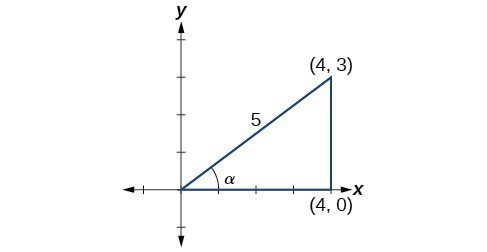Figure 4

Since $\cos \beta =-\frac{5}{13}$ and $\pi <\beta <\frac{3\pi }{2}$, the side adjacent to $\beta$ is $-5$, the hypotenuse is 13, and $\beta$ is in the third quadrant. Again, using the Pythagorean Theorem, we have

$\begin{array}{l}{\left(-5\right)}^{2}+{a}^{2}={13}^{2}\hfill \\ \text{ }25+{a}^{2}=169\hfill \\ {a}^{2}=144\hfill \\ a=\pm 12\hfill \end{array}$

Since $\beta$ is in the third quadrant, $a=-12$.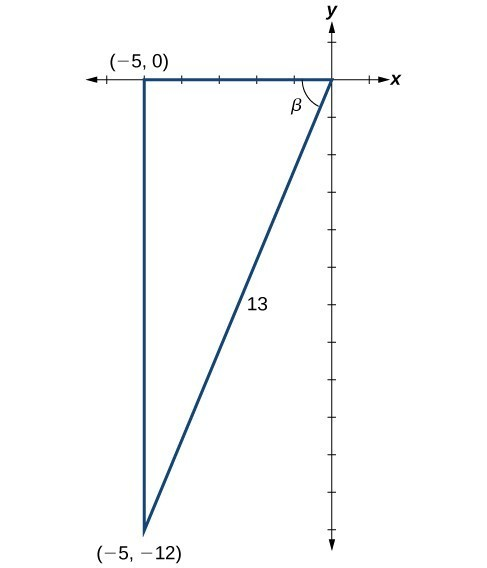Figure 5

The next step is finding the cosine of $\alpha$ and the sine of $\beta$. The cosine of $\alpha$ is the adjacent side over the hypotenuse. We can find it from the triangle in Figure 5: $\cos \alpha =\frac{4}{5}$. We can also find the sine of $\beta$ from the triangle in Figure 5, as opposite side over the hypotenuse: $\sin \beta =-\frac{12}{13}$. Now we are ready to evaluate $\sin \left(\alpha +\beta \right)$.

$\begin{array}{l}\sin \left(\alpha +\beta \right)=\sin \alpha \cos \beta +\cos \alpha \sin \beta \hfill \\ \text{ }=\left(\frac{3}{5}\right)\left(-\frac{5}{13}\right)+\left(\frac{4}{5}\right)\left(-\frac{12}{13}\right)\hfill \\ \text{ }=-\frac{15}{65}-\frac{48}{65}\hfill \\ \text{ }=-\frac{63}{65}\hfill \end{array}$
2. We can find $\cos \left(\alpha +\beta \right)$ in a similar manner. We substitute the values according to the formula.
$\begin{array}{l}\cos \left(\alpha +\beta \right)=\cos \alpha \cos \beta -\sin \alpha \sin \beta \hfill \\ \text{ }=\left(\frac{4}{5}\right)\left(-\frac{5}{13}\right)-\left(\frac{3}{5}\right)\left(-\frac{12}{13}\right)\hfill \\ \text{ }=-\frac{20}{65}+\frac{36}{65}\hfill \\ \text{ }=\frac{16}{65}\hfill \end{array}$
3. For $\tan \left(\alpha +\beta \right)$, if $\sin \alpha =\frac{3}{5}$ and $\cos \alpha =\frac{4}{5}$, then
$\tan \alpha =\frac{\frac{3}{5}}{\frac{4}{5}}=\frac{3}{4}$

If $\sin \beta =-\frac{12}{13}$ and $\cos \beta =-\frac{5}{13}$,
then

$\tan \beta =\frac{\frac{-12}{13}}{\frac{-5}{13}}=\frac{12}{5}$

Then,

$\begin{array}{l}\tan \left(\alpha +\beta \right)=\frac{\tan \alpha +\tan \beta }{1-\tan \alpha \tan \beta }\hfill \\ \text{ }=\frac{\frac{3}{4}+\frac{12}{5}}{1-\frac{3}{4}\left(\frac{12}{5}\right)}\hfill \\ \text{ }=\frac{\text{ }\frac{63}{20}}{-\frac{16}{20}}\hfill \\ \text{ }\text{ }=-\frac{63}{16}\hfill \end{array}$
4. To find $\tan \left(\alpha -\beta \right)$, we have the values we need. We can substitute them in and evaluate.
$\begin{array}{l}\tan \left(\alpha -\beta \right)=\frac{\tan \alpha -\tan \beta }{1+\tan \alpha \tan \beta }\hfill \\ \text{ }=\frac{\frac{3}{4}-\frac{12}{5}}{1+\frac{3}{4}\left(\frac{12}{5}\right)}\hfill \\ \text{ }=\frac{-\frac{33}{20}}{\frac{56}{20}}\hfill \\ \text{ }=-\frac{33}{56}\hfill \end{array}$

### Analysis of the Solution

A common mistake when addressing problems such as this one is that we may be tempted to think that $\alpha$ and $\beta$ are angles in the same triangle, which of course, they are not. Also note that

$\tan \left(\alpha +\beta \right)=\frac{\sin \left(\alpha +\beta \right)}{\cos \left(\alpha +\beta \right)}$

## Use sum and difference formulas for cofunctions

Now that we can find the sine, cosine, and tangent functions for the sums and differences of angles, we can use them to do the same for their cofunctions. You may recall that if the sum of two positive angles is $\frac{\pi }{2}$, those two angles are complements, and the sum of the two acute angles in a right triangle is $\frac{\pi }{2}$, so they are also complements. In Figure 6, notice that if one of the acute angles is labeled as $\theta$, then the other acute angle must be labeled $\left(\frac{\pi }{2}-\theta \right)$.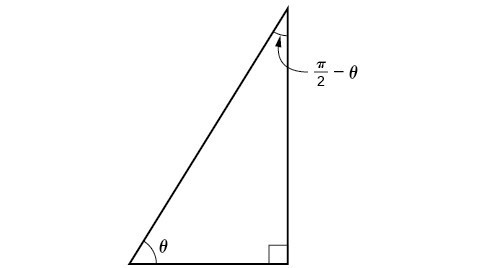Figure 6. From these relationships, the cofunction identities are formed.

Notice also that $\sin \theta =\cos \left(\frac{\pi }{2}-\theta \right):$ opposite over hypotenuse. Thus, when two angles are complimentary, we can say that the sine of $\theta$ equals the cofunction of the complement of $\theta$. Similarly, tangent and cotangent are cofunctions, and secant and cosecant are cofunctions.

### A General Note: Cofunction Identities

The cofunction identities are summarized in the table below.

 $\sin \theta =\cos \left(\frac{\pi }{2}-\theta \right)$ $\cos \theta =\sin \left(\frac{\pi }{2}-\theta \right)$ $\tan \theta =\cot \left(\frac{\pi }{2}-\theta \right)$ $\cot \theta =\tan \left(\frac{\pi }{2}-\theta \right)$ $\sec \theta =\csc \left(\frac{\pi }{2}-\theta \right)$ $\csc \theta =\sec \left(\frac{\pi }{2}-\theta \right)$

Notice that the formulas in the table may also justified algebraically using the sum and difference formulas. For example, using

$\cos \left(\alpha -\beta \right)=\cos \alpha \cos \beta +\sin \alpha \sin \beta$,

we can write

$\begin{array}{l}\cos \left(\frac{\pi }{2}-\theta \right)=\cos \frac{\pi }{2}\cos \theta +\sin \frac{\pi }{2}\sin \theta \hfill \\ \text{ }=\left(0\right)\cos \theta +\left(1\right)\sin \theta \hfill \\ \text{ }=\sin \theta \hfill \end{array}$

### Example 7: Finding a Cofunction with the Same Value as the Given Expression

Write $\tan \frac{\pi }{9}$ in terms of its cofunction.

### Solution

The cofunction of $\tan \theta =\cot \left(\frac{\pi }{2}-\theta \right)$. Thus,

$\begin{array}{l}\tan \left(\frac{\pi }{9}\right)=\cot \left(\frac{\pi }{2}-\frac{\pi }{9}\right)\hfill \\ \text{ }=\cot \left(\frac{9\pi }{18}-\frac{2\pi }{18}\right)\hfill \\ \text{ }=\cot \left(\frac{7\pi }{18}\right)\hfill \end{array}$

### Try It 4

Write $\sin \frac{\pi }{7}$ in terms of its cofunction.

Solution

## Use sum and difference formulas to verify identities

Verifying an identity means demonstrating that the equation holds for all values of the variable. It helps to be very familiar with the identities or to have a list of them accessible while working the problems.

### How To: Given an identity, verify using sum and difference formulas.

1. Begin with the expression on the side of the equal sign that appears most complex. Rewrite that expression until it matches the other side of the equal sign. Occasionally, we might have to alter both sides, but working on only one side is the most efficient.
2. Look for opportunities to use the sum and difference formulas.
3. Rewrite sums or differences of quotients as single quotients.
4. If the process becomes cumbersome, rewrite the expression in terms of sines and cosines.

### Example 8: Verifying an Identity Involving Sine

Verify the identity $\sin \left(\alpha +\beta \right)+\sin \left(\alpha -\beta \right)=2\sin \alpha \cos \beta$.

### Solution

We see that the left side of the equation includes the sines of the sum and the difference of angles.

$\begin{array}{l}\sin \left(\alpha +\beta \right)=\sin \alpha \cos \beta +\cos \alpha \sin \beta \hfill \\ \sin \left(\alpha -\beta \right)=\sin \alpha \cos \beta -\cos \alpha \sin \beta \hfill \end{array}$

We can rewrite each using the sum and difference formulas.

$\begin{array}{l}\sin \left(\alpha +\beta \right)+\sin \left(\alpha -\beta \right)=\sin \alpha \cos \beta +\cos \alpha \sin \beta +\sin \alpha \cos \beta -\cos \alpha \sin \beta \hfill \\ \text{ }=2\sin \alpha \cos \beta \hfill \end{array}$

We see that the identity is verified.

### Example 9: Verifying an Identity Involving Tangent

Verify the following identity.

$\frac{\sin \left(\alpha -\beta \right)}{\cos \alpha \cos \beta }=\tan \alpha -\tan \beta$

### Solution

We can begin by rewriting the numerator on the left side of the equation.

$\begin{array}{ll}\frac{\sin \left(\alpha -\beta \right)}{\cos \alpha \cos \beta }=\frac{\sin \alpha \cos \beta -\cos \alpha \sin \beta }{\cos \alpha \cos \beta }\hfill & \hfill \\ \text{ }=\frac{\sin \alpha \overline{)\cos \beta }}{\cos \alpha \overline{)\cos \beta }}-\frac{\overline{)\cos \alpha }\sin \beta }{\overline{)\cos \alpha }\cos \beta }\begin{array}{cccc}& & & \end{array}\hfill & \text{Rewrite using a common denominator}.\hfill \\ \text{ }=\frac{\sin \alpha }{\cos \alpha }-\frac{\sin \beta }{\cos \beta }\hfill & \text{Cancel}.\hfill \\ \text{ }=\tan \alpha -\tan \beta \hfill & \text{Rewrite in terms of tangent}.\hfill \end{array}$

We see that the identity is verified. In many cases, verifying tangent identities can successfully be accomplished by writing the tangent in terms of sine and cosine.

### Try It 5

Verify the identity: $\tan \left(\pi -\theta \right)=-\tan \theta$.

Solution

### Example 10: Using Sum and Difference Formulas to Solve an Application Problem

Let ${L}_{1}$ and ${L}_{2}$ denote two non-vertical intersecting lines, and let $\theta$ denote the acute angle between ${L}_{1}$ and ${L}_{2}$. Show that

$\tan \theta =\frac{{m}_{2}-{m}_{1}}{1+{m}_{1}{m}_{2}}$

where ${m}_{1}$ and ${m}_{2}$ are the slopes of ${L}_{1}$ and ${L}_{2}$ respectively. (Hint: Use the fact that $\tan {\theta }_{1}={m}_{1}$ and $\tan {\theta }_{2}={m}_{2}$. )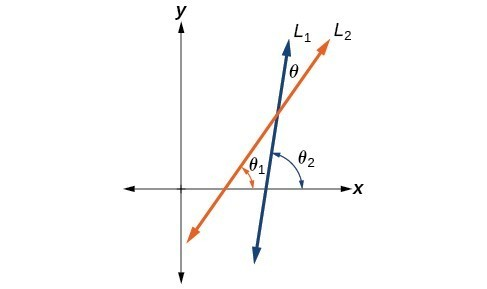Figure 7

### Solution

Using the difference formula for tangent, this problem does not seem as daunting as it might.

$\begin{array}{l}\tan \theta =\tan \left({\theta }_{2}-{\theta }_{1}\right)\hfill \\ \text{ }=\frac{\tan {\theta }_{2}-\tan {\theta }_{1}}{1+\tan {\theta }_{1}\tan {\theta }_{2}}\hfill \\ \text{ }=\frac{{m}_{2}-{m}_{1}}{1+{m}_{1}{m}_{2}}\hfill \end{array}$

### Example 11: Investigating a Guy-wire Problem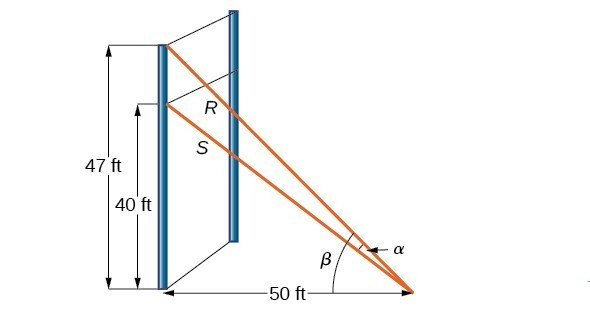Figure 8

For a climbing wall, a guy-wire $R$ is attached 47 feet high on a vertical pole. Added support is provided by another guy-wire $S$ attached 40 feet above ground on the same pole. If the wires are attached to the ground 50 feet from the pole, find the angle $\alpha$ between the wires.

### Solution

Let’s first summarize the information we can gather from the diagram. As only the sides adjacent to the right angle are known, we can use the tangent function. Notice that $\tan \beta =\frac{47}{50}$, and $\tan \left(\beta -\alpha \right)=\frac{40}{50}=\frac{4}{5}$. We can then use difference formula for tangent.

$\tan \left(\beta -\alpha \right)=\frac{\tan \beta -\tan \alpha }{1+\tan \beta \tan \alpha }$

Now, substituting the values we know into the formula, we have

$\begin{array}{l}\text{ }\frac{4}{5}=\frac{\frac{47}{50}-\tan \alpha }{1+\frac{47}{50}\tan \alpha }\hfill \\ 4\left(1+\frac{47}{50}\tan \alpha \right)=5\left(\frac{47}{50}-\tan \alpha \right)\hfill \\ \hfill \end{array}$

Use the distributive property, and then simplify the functions.

$\begin{array}{l}4\left(1\right)+4\left(\frac{47}{50}\right)\tan \alpha =5\left(\frac{47}{50}\right)-5\tan \alpha \hfill \\ 4+3.76\tan \alpha =4.7 - 5\tan \alpha \hfill \\ 5\tan \alpha +3.76\tan \alpha =0.7\hfill \\ 8.76\tan \alpha =0.7\hfill \\ \tan \alpha \approx 0.07991\hfill \\ {\tan }^{-1}\left(0.07991\right)\approx .079741\hfill \end{array}$

Now we can calculate the angle in degrees.

$\alpha \approx 0.079741\left(\frac{180}{\pi }\right)\approx {4.57}^{\circ }$

### Analysis of the Solution

Occasionally, when an application appears that includes a right triangle, we may think that solving is a matter of applying the Pythagorean Theorem. That may be partially true, but it depends on what the problem is asking and what information is given.

## Key Equations

 Sum Formula for Cosine $\cos \left(\alpha +\beta \right)=\cos \alpha \cos \beta -\sin \alpha \sin \beta$ Difference Formula for Cosine $\cos \left(\alpha -\beta \right)=\cos \alpha \cos \beta +\sin \alpha \sin \beta$ Sum Formula for Sine $\sin \left(\alpha +\beta \right)=\sin \alpha \cos \beta +\cos \alpha \sin \beta$ Difference Formula for Sine $\sin \left(\alpha -\beta \right)=\sin \alpha \cos \beta -\cos \alpha \sin \beta$ Sum Formula for Tangent $\tan \left(\alpha +\beta \right)=\frac{\tan \alpha +\tan \beta }{1-\tan \alpha \tan \beta }$ Difference Formula for Tangent $\tan \left(\alpha -\beta \right)=\frac{\tan \alpha -\tan \beta }{1+\tan \alpha \tan \beta }$ Cofunction identities $\begin{array}{l}\sin \theta =\cos \left(\frac{\pi }{2}-\theta \right)\\ \cos \theta =\sin \left(\frac{\pi }{2}-\theta \right)\\ \tan \theta =\cot \left(\frac{\pi }{2}-\theta \right)\\ \cot \theta =\tan \left(\frac{\pi }{2}-\theta \right)\\ \sec \theta =\csc \left(\frac{\pi }{2}-\theta \right)\\ \csc \theta =\sec \left(\frac{\pi }{2}-\theta \right)\end{array}$

## Key Concepts

• The sum formula for cosines states that the cosine of the sum of two angles equals the product of the cosines of the angles minus the product of the sines of the angles. The difference formula for cosines states that the cosine of the difference of two angles equals the product of the cosines of the angles plus the product of the sines of the angles.
• The sum and difference formulas can be used to find the exact values of the sine, cosine, or tangent of an angle.
• The sum formula for sines states that the sine of the sum of two angles equals the product of the sine of the first angle and cosine of the second angle plus the product of the cosine of the first angle and the sine of the second angle. The difference formula for sines states that the sine of the difference of two angles equals the product of the sine of the first angle and cosine of the second angle minus the product of the cosine of the first angle and the sine of the second angle.
• The sum and difference formulas for sine and cosine can also be used for inverse trigonometric functions.
• The sum formula for tangent states that the tangent of the sum of two angles equals the sum of the tangents of the angles divided by 1 minus the product of the tangents of the angles. The difference formula for tangent states that the tangent of the difference of two angles equals the difference of the tangents of the angles divided by 1 plus the product of the tangents of the angles.
• The Pythagorean Theorem along with the sum and difference formulas can be used to find multiple sums and differences of angles.
• The cofunction identities apply to complementary angles and pairs of reciprocal functions.
• Sum and difference formulas are useful in verifying identities.
• Application problems are often easier to solve by using sum and difference formulas.

## Section Exercises

1. Explain the basis for the cofunction identities and when they apply.

2. Is there only one way to evaluate $\cos \left(\frac{5\pi }{4}\right)?$ Explain how to set up the solution in two different ways, and then compute to make sure they give the same answer.

3. Explain to someone who has forgotten the even-odd properties of sinusoidal functions how the addition and subtraction formulas can determine this characteristic for $f\left(x\right)=\sin \left(x\right)$ and $g\left(x\right)=\cos \left(x\right)$. (Hint: $0-x=-x$ )

For the following exercises, find the exact value.

4. $\cos \left(\frac{7\pi }{12}\right)$

5. $\cos \left(\frac{\pi }{12}\right)$

6. $\sin \left(\frac{5\pi }{12}\right)$

7. $\sin \left(\frac{11\pi }{12}\right)$

8. $\tan \left(-\frac{\pi }{12}\right)$

9. $\tan \left(\frac{19\pi }{12}\right)$

For the following exercises, rewrite in terms of $\sin x$ and $\cos x$.

10. $\sin \left(x+\frac{11\pi }{6}\right)$

11. $\sin \left(x-\frac{3\pi }{4}\right)$

12. $\cos \left(x-\frac{5\pi }{6}\right)$

13. $\cos \left(x+\frac{2\pi }{3}\right)$

For the following exercises, simplify the given expression.

14. $\csc \left(\frac{\pi }{2}-t\right)$

15. $\sec \left(\frac{\pi }{2}-\theta \right)$

16. $\cot \left(\frac{\pi }{2}-x\right)$

17. $\tan \left(\frac{\pi }{2}-x\right)$

18. $\sin \left(2x\right)\cos \left(5x\right)-\sin \left(5x\right)\cos \left(2x\right)$

19. $\frac{\tan \left(\frac{3}{2}x\right)-\tan \left(\frac{7}{5}x\right)}{1+\tan \left(\frac{3}{2}x\right)\tan \left(\frac{7}{5}x\right)}$

For the following exercises, find the requested information.

20. Given that $\sin a=\frac{2}{3}$ and $\cos b=-\frac{1}{4}$, with $a$ and $b$ both in the interval $\left[\frac{\pi }{2},\pi \right)$, find $\sin \left(a+b\right)$ and $\cos \left(a-b\right)$.

21. Given that $\sin a=\frac{4}{5}$, and $\cos b=\frac{1}{3}$, with $a$ and $b$ both in the interval $\left[0,\frac{\pi }{2}\right)$, find $\sin \left(a-b\right)$ and $\cos \left(a+b\right)$.

For the following exercises, find the exact value of each expression.

22. $\sin \left({\cos }^{-1}\left(0\right)-{\cos }^{-1}\left(\frac{1}{2}\right)\right)$

23. $\cos \left({\cos }^{-1}\left(\frac{\sqrt{2}}{2}\right)+{\sin }^{-1}\left(\frac{\sqrt{3}}{2}\right)\right)$

24. $\tan \left({\sin }^{-1}\left(\frac{1}{2}\right)-{\cos }^{-1}\left(\frac{1}{2}\right)\right)$

For the following exercises, simplify the expression, and then graph both expressions as functions to verify the graphs are identical.

25. $\cos \left(\frac{\pi }{2}-x\right)$

26. $\sin \left(\pi -x\right)$

27. $\tan \left(\frac{\pi }{3}+x\right)$

28. $\sin \left(\frac{\pi }{3}+x\right)$

29. $\tan \left(\frac{\pi }{4}-x\right)$

30. $\cos \left(\frac{7\pi }{6}+x\right)$

31. $\sin \left(\frac{\pi }{4}+x\right)$

32. $\cos \left(\frac{5\pi }{4}+x\right)$

For the following exercises, use a graph to determine whether the functions are the same or different. If they are the same, show why. If they are different, replace the second function with one that is identical to the first. (Hint: think $2x=x+x$.
)

33. $f\left(x\right)=\sin \left(4x\right)-\sin \left(3x\right)\cos x,g\left(x\right)=\sin x\cos \left(3x\right)$

34. $f\left(x\right)=\cos \left(4x\right)+\sin x\sin \left(3x\right),g\left(x\right)=-\cos x\cos \left(3x\right)$

35. $f\left(x\right)=\sin \left(3x\right)\cos \left(6x\right),g\left(x\right)=-\sin \left(3x\right)\cos \left(6x\right)$

36. $f\left(x\right)=\sin \left(4x\right),g\left(x\right)=\sin \left(5x\right)\cos x-\cos \left(5x\right)\sin x$

37. $f\left(x\right)=\sin \left(2x\right),g\left(x\right)=2\sin x\cos x$

38. $f\left(\theta \right)=\cos \left(2\theta \right),g\left(\theta \right)={\cos }^{2}\theta -{\sin }^{2}\theta$

39. $f\left(\theta \right)=\tan \left(2\theta \right),g\left(\theta \right)=\frac{\tan \theta }{1+{\tan }^{2}\theta }$

40. $f\left(x\right)=\sin \left(3x\right)\sin x,g\left(x\right)={\sin }^{2}\left(2x\right){\cos }^{2}x-{\cos }^{2}\left(2x\right){\sin }^{2}x$

41. $f\left(x\right)=\tan \left(-x\right),g\left(x\right)=\frac{\tan x-\tan \left(2x\right)}{1-\tan x\tan \left(2x\right)}$

For the following exercises, find the exact value algebraically, and then confirm the answer with a calculator to the fourth decimal point.

42. $\sin \left({75}^{\circ }\right)$

43. $\sin \left({195}^{\circ }\right)$

44. $\cos \left({165}^{\circ }\right)$

45. $\cos \left({345}^{\circ }\right)$

46. $\tan \left(-{15}^{\circ }\right)$

For the following exercises, prove the identities provided.

47. $\tan \left(x+\frac{\pi }{4}\right)=\frac{\tan x+1}{1-\tan x}$

48. $\frac{\tan \left(a+b\right)}{\tan \left(a-b\right)}=\frac{\sin a\cos a+\sin b\cos b}{\sin a\cos a-\sin b\cos b}$

49. $\frac{\cos \left(a+b\right)}{\cos a\cos b}=1-\tan a\tan b$

50. $\cos \left(x+y\right)\cos \left(x-y\right)={\cos }^{2}x-{\sin }^{2}y$

51. $\frac{\cos \left(x+h\right)-\cos x}{h}=\cos x\frac{\cos h - 1}{h}-\sin x\frac{\sin h}{h}$

For the following exercises, prove or disprove the statements.

52. $\tan \left(u+v\right)=\frac{\tan u+\tan v}{1-\tan u\tan v}$

53. $\tan \left(u-v\right)=\frac{\tan u-\tan v}{1+\tan u\tan v}$

54. $\frac{\tan \left(x+y\right)}{1+\tan x\tan x}=\frac{\tan x+\tan y}{1-{\tan }^{2}x{\tan }^{2}y}$

55. If $\alpha ,\beta$, and $\gamma$ are angles in the same triangle, then prove or disprove $\sin \left(\alpha +\beta \right)=\sin \gamma$.

57. If $\alpha ,\beta$, and $y$ are angles in the same triangle, then prove or disprove $\tan \alpha +\tan \beta +\tan \gamma =\tan \alpha \tan \beta \tan \gamma$